# 《2019战略十问》系列之六：竞争的基本单位是什么？➢ 喜之郎=果冻

➢ 格力=空调

➢ 康师傅=方便面

➢ 百度=中文搜索

➢ 万科=地产

➢ 王老吉=凉茶

➢ 天猫=品牌电商

➢ 唯品会=特价电商

➢ 瓜子=二手车直卖网

➢ 星巴克=美式咖啡馆

➢ 红牛=功能饮料

➢ 豆本豆=豆奶

➢ 六个核桃=核桃乳

➢ 奔驰=尊贵

➢ 宝马=驾趣

➢ 沃尔沃=安全

➢ 海飞丝=去屑

➢ 飘柔=柔顺

➢ 潘婷=营养

➢ 芝华仕=功能沙发

➢ 方太=高端厨电

➢ 老板=大吸力油烟机

➢ 简一=大理石瓷砖

➢ TATA=静音木门

... ...

[责任编辑：周凡]

•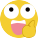好文
•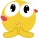钦佩
•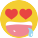喜欢
•泪奔
•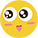可爱
•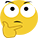思考